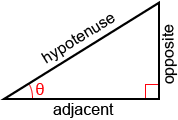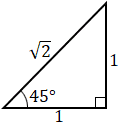# Trigonometry

Trigonometry is a branch of mathematics that concerns the relationships between the sides and angles of triangles. There are three main trigonometric functions: sine, cosine, and tangent.

## Right triangle

Right triangles are widely used in trigonometry. A right triangle is a triangle in which one angle has a measurement of 90° (a right angle), such as the triangle shown below.The other angles of a right triangle are often represented by Greek letters, such as θ, α, and β. The sides of a right triangle are referenced as follows:

• Adjacent: the side next to the angle
• Opposite: the side opposite the angle
• Hypotenuse: the side opposite the right angle. It is also the longest side.

## Trigonometric functions

The main trigonometric functions are sine, cosine, and tangent. Sine, cosine, and tangent are often abbreviated as sin, cos, and tan. Trigonometric functions are also called circular functions.Example:What is the value of sin(45°), cos(45°), and tan(45°)?Example:Bob walked 300 m straight up on a 30° hill, how high did Bob climb?

 Height = distance walked × sin(30°) = 300 × 0.5 = 150 m

Thus, Bob reached a height of 150 m after walking 300 m up the slope of the hill.

Secant, cotangent, and cosecant are also trigonometric functions, but they are rarely used.## Inverse trigonometric functions

The inverse trigonometric functions are the inverse functions of the trigonometric functions:

 Given y = sine(θ), θ = arcsin(y) Given y = cosine(θ), θ = arccos(y) Given y = tangent(θ), θ = arctan(y)

Arcsin, arccos, and arctan are the inverse functions of sine, cosine, and tangent respectively.

Example:Given a right triangle with opposite length 3 and hypotenuse length 6, what is the value of θ?## Non-right triangles

Unlike right triangles, the sine, cosine, and tangent of a non-right triangle cannot be used to calculate the length of the sides and angles of the triangle directly. Instead the following rules can be used.### The sine rule

For an arbitrary triangle,The sine rule can be used when one side and any two angles are known.

Example:

Given, a=3, B=70°, C=45°, find A, b, and c,

 A = 180° - B - C = 180° - 70° - 45° = 65°### The cosine rule

For an arbitrary triangle,

 a2 = b2 + c2 – 2bc·cos(A) b2 = a2 + c2 – 2ac·cos(B) c2 = a2 + b2 – 2ab·cos(C)

The cosine rule can be used when one angle and the two sides next to it are known.

Example:

Given, A=45°, b=3, c=5, find a,

 a2 = b2 + c2 – 2bc·cos(A) = 32 + 52 + 2×3×5×cos(45°) = 55.213